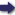(London :  Kegan Paul, Trench, Trübner & Co.,  1910.)

 Tools

## Search this bookPrev Page 34 Next``` 34 ALBERUNTS INDIA. The ahao-- of Ya'kub Ibn Tarik in the calculation of the universal given by solar and unardtra days. As he translated from the T4rik. Indian language a calculation the reasons of which he did not understand, it would have been his duty to examine it, and to check the various numbers of it one by the other. He mentions in his book also the method of ahargana, i.e. the resolution of years, but his descrip¬ tion is not correct; for he says :— " Multiply the months of the given number of years by the number of the adhimdsa months which have elapsed up to the time in question, according to the well-known rules of adhimdsa. Divide the product by the solar months. The quotient is the number of complete adhimdsa months plus its fractions which have elapsed up to the date in question." The mistake is here so evident that even a copyist would notice it; how much more a mathematician who makes a computation according to this method; for he multiplies by the partial adhimdsa instead of the universal. A second Bcsides, Ya'kiib mentions in his book another and given by perfectly correct method of resolution, which is this: "When you have found the number of months of the years, multiply them by the number of the lunar months, and divide the product by the solar months. The quotient is the number of adhimdsa months to¬ gether with the number of the months of the years in question. " This number you multiply by 30, and you add to the product the days which have elapsed of the current month. The sum represents the lunar days. " If, instead of this, the first number of months were multiplied by 30, and the past portion of the month were added to the product, the sum would represent the partial solar days; and if this number were further computed according to the preceding method, we should get the adhimdsa, days together with the solar days." Ya'kub. ```Prev Page 34 Next